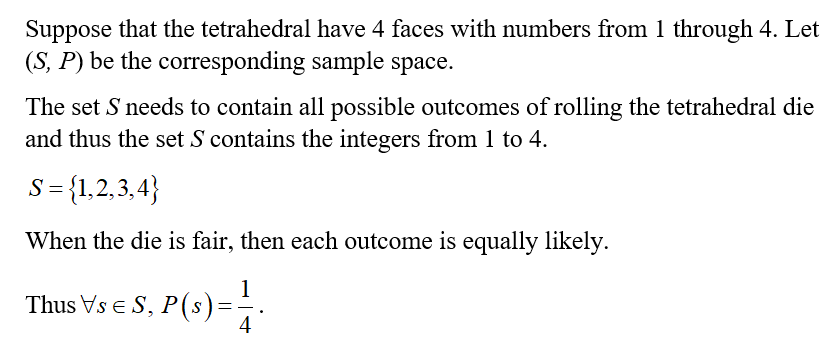# Tetrahedral dice.  A tetrahedron is a solid figure with four faces, each of which is an equilateral triangle.  We can make dice in the shape of tetrahedra and label their faces with the numbers 1 through 4.  When such a die is rolled, the number that lands face down on the table is the result.Create a sample space that represents the toss of a tetrahedral die.Create a sample space that represents the toss of a pair of tetrahedral dice.

Question
61 views

Tetrahedral dice.  A tetrahedron is a solid figure with four faces, each of which is an equilateral triangle.  We can make dice in the shape of tetrahedra and label their faces with the numbers 1 through 4.  When such a die is rolled, the number that lands face down on the table is the result.

1. Create a sample space that represents the toss of a tetrahedral die.
2. Create a sample space that represents the toss of a pair of tetrahedral dice.
check_circle

Step 1

1. Create a sample space that represents the toss of a tetrahedral die as follows....

### Want to see the full answer?

See Solution

#### Want to see this answer and more?

Solutions are written by subject experts who are available 24/7. Questions are typically answered within 1 hour.*

See Solution
*Response times may vary by subject and question.
Tagged in

### Math